RRB NTPC Mock Test - 5 (English)

# RRB NTPC Mock Test - 5 (English)

Test Description

## 100 Questions MCQ Test RRB NTPC Mock Tests Series | RRB NTPC Mock Test - 5 (English)

RRB NTPC Mock Test - 5 (English) for Railways 2022 is part of RRB NTPC Mock Tests Series preparation. The RRB NTPC Mock Test - 5 (English) questions and answers have been prepared according to the Railways exam syllabus.The RRB NTPC Mock Test - 5 (English) MCQs are made for Railways 2022 Exam. Find important definitions, questions, notes, meanings, examples, exercises, MCQs and online tests for RRB NTPC Mock Test - 5 (English) below.
Solutions of RRB NTPC Mock Test - 5 (English) questions in English are available as part of our RRB NTPC Mock Tests Series for Railways & RRB NTPC Mock Test - 5 (English) solutions in Hindi for RRB NTPC Mock Tests Series course. Download more important topics, notes, lectures and mock test series for Railways Exam by signing up for free. Attempt RRB NTPC Mock Test - 5 (English) | 100 questions in 90 minutes | Mock test for Railways preparation | Free important questions MCQ to study RRB NTPC Mock Tests Series for Railways Exam | Download free PDF with solutions
 1 Crore+ students have signed up on EduRev. Have you?
RRB NTPC Mock Test - 5 (English) - Question 1

### Which among the following is not an ore of Aluminium (Al)?

Detailed Solution for RRB NTPC Mock Test - 5 (English) - Question 1
• The ores of Aluminium are - Bauxite, Corundum, Felspar, Cryolite, Alunite and Kaolin.
• Chalcocite is an important copper mineral ore
• The process of extracting metal in pure form from its ore is known as metallurgy.
RRB NTPC Mock Test - 5 (English) - Question 2

### Indian railways launched the Rail MADAD mobile app in June 2018. Expand MADAD.

Detailed Solution for RRB NTPC Mock Test - 5 (English) - Question 2
• MADAD stands for Mobile Application for Desired Assistance During travel.
• The app was launched by Railway Minister Piyush Goyal along with other app named "Menu on Rails".
• The apps were launched to ease the travelling experience of the passengers.
• The aim of Rail MADAD app is to expedite and streamline passenger complaints redressal.
• The aim of Menu on Rails is to provide the list of meals and beverages available in trains with their prices.
RRB NTPC Mock Test - 5 (English) - Question 3

### Which of the following satellite is known as the "Big bird"?

Detailed Solution for RRB NTPC Mock Test - 5 (English) - Question 3
• The satellite GSAT-11 is known as the "Big bird". It is the heaviest (5,854 kg) satellite of India.
• The ISRO's satellite was launched by Ariane 5 rocket in December 2018 from the French space port of Kourou in South America.
• The Hyper-Spectral Imaging Satellite (HysIS) was successfully launched by PSLV-C43 in November 2018, from Satish Dhawan Space Centre (SDSC) in Sriharikota.
• GSAT-29 launched by ISRO in November 2018, is a communication satellite to improve the communication networks, specifically in the Jammu and Kashmir and North East.
• Microsat-R is a defence satellite for the Defence Research and Development Organisation (DRDO) launched by the PSLV.
RRB NTPC Mock Test - 5 (English) - Question 4

Who is the captain of the Hong Kong cricket team?

Detailed Solution for RRB NTPC Mock Test - 5 (English) - Question 4
• Anshuman Rath is the captain of the Hong Kong cricket team.
• The team won the 2018 Asia Cup Qualifier under his captainship. Hong Kong defeated UAE.
• 2018 Asia Cup was won by India, defeating Bangladesh.
• William Porterfield is the captain of Ireland cricket team.
• Kyle Coetzer is the captain of Scotland cricket team.
• Paras Khadka is the captain of the Nepal team
RRB NTPC Mock Test - 5 (English) - Question 5

Which type of lens is used by persons suffering from Hypermetropia?

Detailed Solution for RRB NTPC Mock Test - 5 (English) - Question 5
• Persons suffering Hypermetropia use Convex lens.
• Hypermetropia, also known as far sightedness, is the disorder in which a person cannot see the nearby objects clearly but can see the distant objects clearly.
• Concave lens are used for Myopia, in which person can see the nearby objects but fail to see the distant objects.
• Bifocal lens are used in case of Presbyopia, in which power of accommodation of the eye decreases. In bifocal lenses the upper portion contains the concave lens, while the lower part contains the convex lens.
• Convexo-concave lens is a type of concave lens in which one side is bulged out (which forms the convex side) and the other side is concave, which is thin in the middle and thick at the edges.
RRB NTPC Mock Test - 5 (English) - Question 6

Atomic mass unit is defined in terms of which of the following?

Detailed Solution for RRB NTPC Mock Test - 5 (English) - Question 6
• Atomic mass unit is defined in terms of carbon-12 (C-12).
• The atomic mass unit is used to express atomic and molecular weights and it is equal to one-twelfth of the mass of the one C-12 atom.
• The atomic weight of the hydrogen atom is used to compare the atomic weight of other elements.
• N-15 is the non-radioactive, stable isotope of nitrogen. N-13, N-14, N-15, etc. are the other isotopes of nitrogen.
• C-14 is an radioactive isotope of carbon used in carbon dating (determining the age of carbonaceous materials). C-12, C-13, and C-14 are the isotopes of carbon.
RRB NTPC Mock Test - 5 (English) - Question 7

The study of grass is known as ________.

Detailed Solution for RRB NTPC Mock Test - 5 (English) - Question 7
• The study of grass is known as Agrostology.
• The study of soil management and the production of crop plants is known as Agronomy.
• The study of medicinal plants is known as Pharmacognosy.
• The study of shrubs and trees is known as Dendrology.
RRB NTPC Mock Test - 5 (English) - Question 8

Which of the martial art dance form belongs to the Punjab region of India?

Detailed Solution for RRB NTPC Mock Test - 5 (English) - Question 8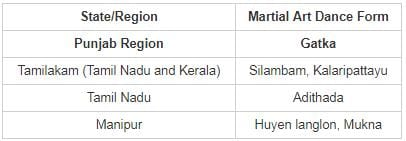RRB NTPC Mock Test - 5 (English) - Question 9

In the following question, by using which mathematical operators will the expression become correct.

49_7_6_7

Detailed Solution for RRB NTPC Mock Test - 5 (English) - Question 9

Let us check for all option,

1) 49 > 7 > 6 ≥ 7, False

2) 49 = 7 + 6 + 7, False

3) 49 = 7 + 6 - 7, False

4) 49 = 7 × 6 + 7, True

Hence, "=, ×, +” is the correct set of sign.

RRB NTPC Mock Test - 5 (English) - Question 10

Which of the following hydrocarbon is termed as "Fruit ripening gas"?

Detailed Solution for RRB NTPC Mock Test - 5 (English) - Question 10
• Ethylene is known as the "Fruit ripening gas".
• The chemical formula of Ethylene (Ethene) is C2H4.
• It is the simplest alkene (compounds containing carbon-carbon double bond).
• Methane (CH4), the simplest alkane (compounds containing carbon-carbon single bond), is known as Marsh gas.
• Propane is an alkane containing 3 carbon atoms (chemical formula - C3H8)
RRB NTPC Mock Test - 5 (English) - Question 11

Which of the following is a protozoan?

Detailed Solution for RRB NTPC Mock Test - 5 (English) - Question 11
• Trypanosoma is a protozoan. It is the causative pathogen for African Sleeping Sickness.
• Sycon belongs to phylum Porifera.
• Fasciola belongs to phylum Platyhelminthes. It is known as "sheep liver fluke" as it causes disease in sheep and cattle.
• Nereis belongs to phylum Annelida.
RRB NTPC Mock Test - 5 (English) - Question 12

Six friends Ajay, Goarav, Adharav, Saurav, Pari, and Kashvi are sitting in two parallel rows containing three people each. Ajay, Goarav and Adharav are sitting in row-1 facing north and Saurav, Pari and Kashvi are sitting in row-2 facing south (but not necessarily in the same order). Thus, each person sitting in row-1 face another person sitting in row-2. Ajay, Goarav, Saurav and Pari are sitting at extreme ends. Saurav do not face Goarav. Ajay is sitting at extreme right end.

Who is sitting at extreme right of row-2?

Detailed Solution for RRB NTPC Mock Test - 5 (English) - Question 12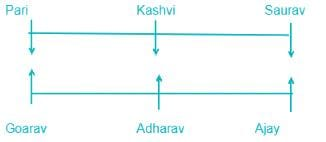Note: Left and right end depend on the direction of the people. If people are facing North then the left end will be in the west and the right end will be in East but if people are facing South then left end for them will be in East and right end will be West.

Persons in row 2 are facing South. Hence the right end of row 2 is opposite to the right end of the persons of row 1 who are facing North.

Hence, Pari is sitting at the extreme right of row-2.

RRB NTPC Mock Test - 5 (English) - Question 13

Direction: Study the following figure and answer the questions given below.
Where, rectangle represent Artists, Circle represent singers and Triangle represents Doctors.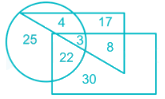Q. How many doctors are neither artists nor singers?

Detailed Solution for RRB NTPC Mock Test - 5 (English) - Question 13

The section of the triangle that neither intersect with rectangle nor with circle represents the doctors that are neither artists nor singers.
The number of doctors who are neither artists nor singers is 17.

RRB NTPC Mock Test - 5 (English) - Question 14

Direction: Study the following figure and answer the questions given below.
Where, rectangle represent Artists, Circle represent singers and Triangle represents Doctors.Q. Refer to the above question and answer how many doctors are both singers and artists?

Detailed Solution for RRB NTPC Mock Test - 5 (English) - Question 14

The section of the triangle that intersect with both rectangle and circle represents the doctors that are both artists and singers.
The number of doctors who are both singers and artists is 3.

RRB NTPC Mock Test - 5 (English) - Question 15

Which of the following is/are effects of Radioactive pollution?

Detailed Solution for RRB NTPC Mock Test - 5 (English) - Question 15
• Radioactive pollution can occur due to two reasons as natural and artificial. UV and IR radiations are natural radiations whereas X-rays and radiations from atomic energy plants are artificial radiations.
• Chernobyl, Windscale, and Three Miles Island mishaps are the major mishaps of the world to the present. Thousands of people have been affected for the long term due to these accidents.
• Some of the effects of radiations are as follows-
1. Cancerous ulceration occurs due to higher radiations of X-rays.
2. Tissues in the body are destroyed.
3. Genetic changes occur.
4. Vision is adversely affected.
RRB NTPC Mock Test - 5 (English) - Question 16

Which of the following jumble words is not the name of the major gases present in Air?

Detailed Solution for RRB NTPC Mock Test - 5 (English) - Question 16

1) TRONIENG = NITROGEN
2) YGENOX = OXYGEN
3) GONRA = ARGON
4) LFIEDIEQU PEROELMUT = LIQUEFIED PETROLEUM (it is not a gas present in air)
Hence LFIEDIQU PEROELMUT is the correct answer.

RRB NTPC Mock Test - 5 (English) - Question 17

Direction: Study the following information carefully and answer the question.
A said to B,” A person has to walk 6 km to the south of the point T to reach my house. From point Q one has to walk 10km towards north and then turn 45 degree towards west and walk 6km to reach point T.”

Q. What is the total distance between T and Q?

Detailed Solution for RRB NTPC Mock Test - 5 (English) - Question 17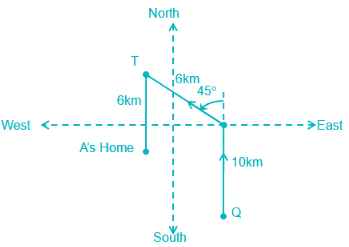RRB NTPC Mock Test - 5 (English) - Question 18

Direction: Study the following information carefully and answer the question.
A said to B,” A person has to walk 6 km to the south of the point T to reach my house. From point Q one has to walk 10km towards north and then turn 45 degree towards west and walk 6km to reach point T.”

Q. In which direction is A’s house with respect to point Q?

Detailed Solution for RRB NTPC Mock Test - 5 (English) - Question 18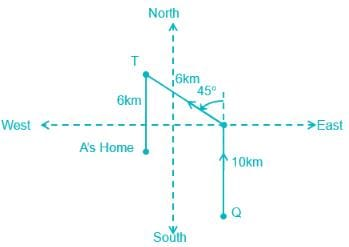RRB NTPC Mock Test - 5 (English) - Question 19

Direction: Read the following information carefully and answer the question that follows:
There are six persons Dheer, Dennise, Dev, Dharmesh, Daniel and David, who got different ranks in an exam. David’s rank was immediately after Dev. Dev’s rank is better than all of them. Dheer got the poorest rank among all. Dharmesh got better rank than three of them. Daniel got a bettar rank than Dennise.

Q. Who got the fifth least rank?

Detailed Solution for RRB NTPC Mock Test - 5 (English) - Question 19

1) David’s rank was immediately after Dev. Dev’s rank is better than all of them.

Dev > David > ….. > ….. > ….. > ……

2) Dheer got the poorest rank among them all.

Dev > David > ….. > ….. > ….. > Dheer

3) Dharmesh got better rank than three of them.

Dev > David > Dharmesh > ….. > ….. > Dheer

4) Daniel got a bettar rank than Dennise.

Dev > David > Dharmesh > Daniel > Dennise > Dheer

Note: Dheer got the least rank, Dennise got second least rank, Daniel got third least rank, Dharmesh got fourth least rank, David got fifth least rank and Dev got sixth least rank.​

RRB NTPC Mock Test - 5 (English) - Question 20

What is pH of Lemon Juice?

Detailed Solution for RRB NTPC Mock Test - 5 (English) - Question 20

pH of some common aqueous solutions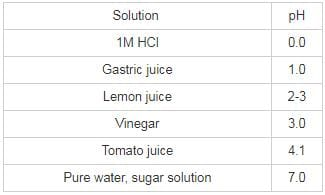RRB NTPC Mock Test - 5 (English) - Question 21

Recently India tested a new indigenous air-launched missile called NGARM. What is strike range of NGARM?

Detailed Solution for RRB NTPC Mock Test - 5 (English) - Question 21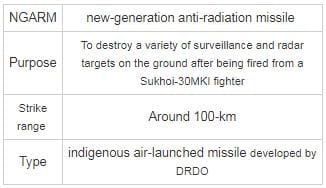RRB NTPC Mock Test - 5 (English) - Question 22

All the six members of a family A, B, C, D, E and F are travelling together. B is the son of C but C is not the mother of B. A and C are a married couple. E is the brother of C. D is the daughter of A. F is the brother of B.

Q. How is F’s mother related to daughter of C?

Detailed Solution for RRB NTPC Mock Test - 5 (English) - Question 22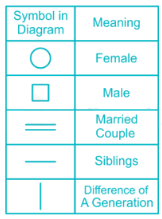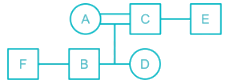F’s mother is A.

Daughter of C is D.

Thus, A is mother of D.

Hence, the correct answer is mother.

RRB NTPC Mock Test - 5 (English) - Question 23

Pointing to a lady in the photograph, Jaya said, “Her son’s father is the son-in-law of my mother.” How is Jaya related to the lady?

Detailed Solution for RRB NTPC Mock Test - 5 (English) - Question 23

From the given information,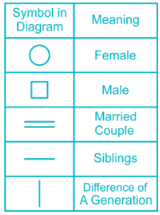Based on given data, we can draw family tree-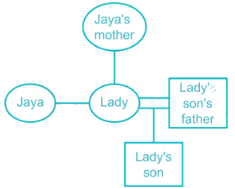Lady’s son’s father is the husband of the lady.

The husband of the lady is the son-in-law of Jaya’s mother.

So, the lady is the daughter of Jaya’s mother.

Thus, Jaya is the sister of the Lady.

RRB NTPC Mock Test - 5 (English) - Question 24

Who among the following recently appointed as Chairman of ISRO?

Detailed Solution for RRB NTPC Mock Test - 5 (English) - Question 24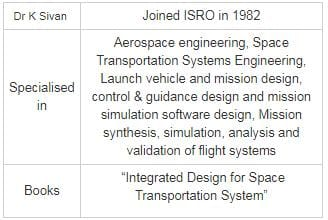RRB NTPC Mock Test - 5 (English) - Question 25

Which of the following Mission will be launched by ISRO to study the Sun?

Detailed Solution for RRB NTPC Mock Test - 5 (English) - Question 25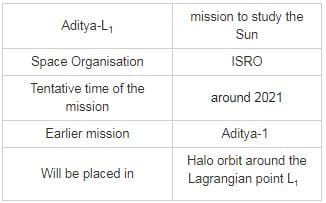RRB NTPC Mock Test - 5 (English) - Question 26

Direction: In a certain code language ‘festival for woman only’ is written as ’77 78 79 80’, ‘provide peace to woman’ is written as ’81 82 83 78’, ‘woman like to celebrate’ is written as ’78 85 86 81’ and ‘celebrate peace in festival’ is written as ’82 79 87 85’.

Q. What may the possible code for ‘provide idea’ in the given code language?

Detailed Solution for RRB NTPC Mock Test - 5 (English) - Question 26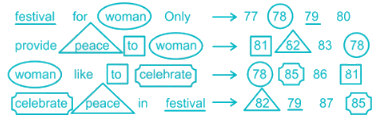Code for provide is 83

Code for idea is not given, therefore we will check all the options:

1) 86 89 ⇒ False (code of provide i.e. 83 is not present)

2) 80 79  ⇒ False  (code  of  provide  i.e. 83 is not present)

3) 89 83 ⇒ True (83 for provide and 89 for idea is possible because 89 is not given in the above code)

4) 83 80 ⇒ False (Provide is coded as 83 and either for or only is coded as 80)

RRB NTPC Mock Test - 5 (English) - Question 27

In a certain code language, GAME is written as FHZBLNDF then BLUE will be?

Detailed Solution for RRB NTPC Mock Test - 5 (English) - Question 27

The pattern for the code is as follows,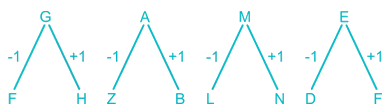Each letter of the given word is replaced by its adjacent letters.

Similarly,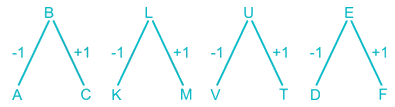Therefore, BLUE is coded as ACKMTVDF.

So, the answer is option (3).

RRB NTPC Mock Test - 5 (English) - Question 28

Find the wrong term in the series.

AA, BD, CI, DR, EY

Detailed Solution for RRB NTPC Mock Test - 5 (English) - Question 28

The pattern followed is:

A = 1 so, 12 = 1 so, 1 = A.

B = 2 so, 22 = 4 so, 4 = D.

C = 3 so, 32 = 9 so, 9 = I.

D = 4 so, 42 = 16 so, 16 = P ≠ R

E = 5 so, 52 = 25 so, 25 = Y.

So, the wrong term is DR.

RRB NTPC Mock Test - 5 (English) - Question 29

A series is given with one term missing. Select the correct alternative from the given ones that will complete the series.

8, 13, 23, 38, 58, 83, ?

Detailed Solution for RRB NTPC Mock Test - 5 (English) - Question 29

The pattern followed here is:

8 + 5 = 13

13 + 10 = 23

23 + 15 = 38

38 + 20 = 58

58 + 25 = 83

Similarly,

83 + 30 = 113

Hence, 113 is a term which will complete the series.

RRB NTPC Mock Test - 5 (English) - Question 30

Out of the four given figures, three figures are similar in a certain way, one figure is not like the others. That means three figures form a group based on some common properties. Find the figure which does not belong to the group.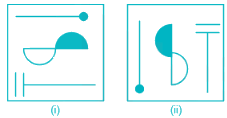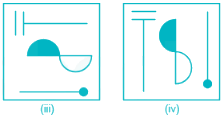Detailed Solution for RRB NTPC Mock Test - 5 (English) - Question 30

Image (i): the shaded portion of the central semi-circle is in west direction while the = sign bar is in West direction.

Image (ii): the shaded portion of the central semi-circle and the = sign bar are in North direction.

Image (iii): the shaded portion of the central semi-circle and the = sign bar are in West direction.

Image (iv): the shaded portion of the central semi-circle and the = sign bar are in North direction.

In all other figures, shaded portion of the central semi-circle and the = sign bar come on the same side except for figure (i).

Hence, figure (i) is the correct answer.

RRB NTPC Mock Test - 5 (English) - Question 31

Select the related word from the given alternatives.
Pratibha Patil : First Woman President of India : : C.D.Deshmukh : ?

Detailed Solution for RRB NTPC Mock Test - 5 (English) - Question 31

As Pratibha Patil was the first woman president of India. Similarly, C.D.Deshmukh was the first Indian Governor of RBI.

RRB NTPC Mock Test - 5 (English) - Question 32

In the following question find out the alternative which will replace the question mark.
Grapes : Vineyard : : Birds : ?

Detailed Solution for RRB NTPC Mock Test - 5 (English) - Question 32

Vineyard is a place for grape plantation,

Similarly, Aviary is a place for keeping birds.

Hence, the answer is Aviary.

RRB NTPC Mock Test - 5 (English) - Question 33

In the following question, select the odd word from the given alternatives.

Detailed Solution for RRB NTPC Mock Test - 5 (English) - Question 33

All except Ounce is the measure of length, but Ounce is measure of weight

Hence, Ounce is odd word.

RRB NTPC Mock Test - 5 (English) - Question 34

'Naseem-Al-Bahr' is bilateral naval exercise between India and ________.

Detailed Solution for RRB NTPC Mock Test - 5 (English) - Question 34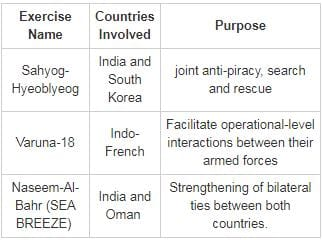RRB NTPC Mock Test - 5 (English) - Question 35

Bihu is folk dance from which of the following Indian state?

Detailed Solution for RRB NTPC Mock Test - 5 (English) - Question 35

The Bihu dance is a folk dance from the Indian state of Assam related to the Bihu festival and an important part of Assamese culture.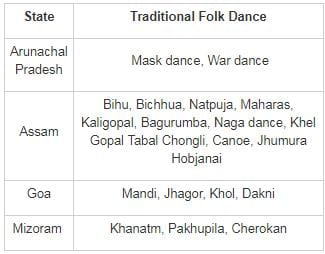RRB NTPC Mock Test - 5 (English) - Question 36

FIFA Football World Cup of 2022 will be hosted by ________.

Detailed Solution for RRB NTPC Mock Test - 5 (English) - Question 36

FIFA World Cup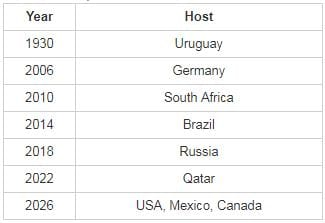RRB NTPC Mock Test - 5 (English) - Question 37

The official seal of the Government of India is based on the capital or pillar head of the Ashoka pillar at ________.

Detailed Solution for RRB NTPC Mock Test - 5 (English) - Question 37
• The official seal of the Government of India is based on the capital or pillar head of the Ashoka pillar at Sarnath. The Ashoka pillar has four lions. On a horizontal strip below each lion, there is a wheel or chakra.
• We can view only one of these chakras at a time. There is a horse on one side and a bull on the other side of the chakra. Similarly, on the side that is not in view on the seal, there is an elephant and a lion on either side of the chakra.
RRB NTPC Mock Test - 5 (English) - Question 38

Who among the following is known as the Father of Blood Circulation?

Detailed Solution for RRB NTPC Mock Test - 5 (English) - Question 38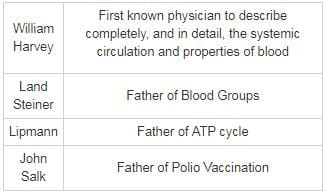RRB NTPC Mock Test - 5 (English) - Question 39

Recently which country changed its political capital from Bujumbura to Gitega?

Detailed Solution for RRB NTPC Mock Test - 5 (English) - Question 39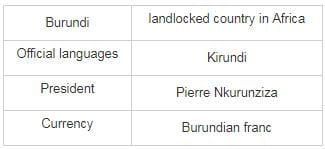RRB NTPC Mock Test - 5 (English) - Question 40

Who received Man Booker International Prize in 2018 for his/her work 'Flights'?

Detailed Solution for RRB NTPC Mock Test - 5 (English) - Question 40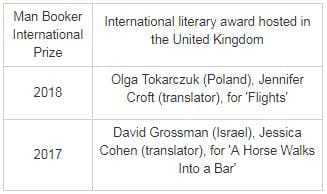RRB NTPC Mock Test - 5 (English) - Question 41

Who among the following Indian female cricket players is first to complete 2000 runs in T-20 cricket?

Detailed Solution for RRB NTPC Mock Test - 5 (English) - Question 41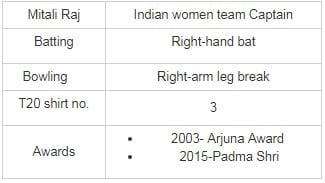RRB NTPC Mock Test - 5 (English) - Question 42

‘Lincoln in the Bardo’ is a book written by ________.

Detailed Solution for RRB NTPC Mock Test - 5 (English) - Question 42

‘Lincoln in the Bardo’ is a book written by George Saunders.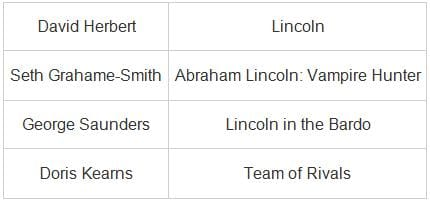RRB NTPC Mock Test - 5 (English) - Question 43

What is the SI unit of self inductance?

Detailed Solution for RRB NTPC Mock Test - 5 (English) - Question 43

Self-inductance is the phenomenon by which an opposing induced e.m.f. is produced in a coil due to change in the magnetic flux or currently associated with the coil.

We know,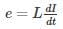(No negative sign as taking the only magnitude into consideration)

where e is the induced e.m.f.

dI/dt is the rate of change of current in the coil at that instant

L is the self-inductance
or, L=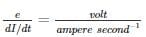= V −sec/A = Henry(H)

RRB NTPC Mock Test - 5 (English) - Question 44

When was the 30th Road Safety Week 2019 observed in india?

Detailed Solution for RRB NTPC Mock Test - 5 (English) - Question 44

30th Road Safety Week 2019 was celebrated in India from 4th Feb to 10th Feb. There had been various awareness campaigns and events which were conducted across the country in all the states. The theme was “Sadak Suraksha Jeevan Raksha”.

RRB NTPC Mock Test - 5 (English) - Question 45

Which of the following does resistivity NOT depend on?

Detailed Solution for RRB NTPC Mock Test - 5 (English) - Question 45
• Unlike resistance, the value of resistivity is independent of the dimensions of the conductor.
• Resistivity is inversely proportional to the number of free electrons per unit volume of the conductor and to the average relaxation time of the free electrons in the conductor.
• Resistivity is affected by the nature of the material of the conductor. Also, it is directly proportional to the temperature of the conductor, that is, it increases with the increase in temperature.
RRB NTPC Mock Test - 5 (English) - Question 46

If ‘+‘ means ‘÷’, ‘-‘ means ‘×‘, ‘÷‘ means ‘+‘ and ‘×’ means ‘-‘, then what is the value of 33 + 3 - 8 + 4 ÷ 10 × 2 × 5?

Detailed Solution for RRB NTPC Mock Test - 5 (English) - Question 46

Given Equation: 33 + 3 - 8 + 4 ÷ 10 × 2 × 5

Changing operators as given format.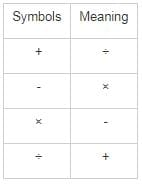Given expression: 33 + 3 - 8 + 4 ÷ 10 × 2 × 5

After interchanging symbols: 33 ÷ 3 × 8 ÷ 4 + 10 - 2 - 5

= 33 ÷ 3 × 8 ÷ 4 + 10 - 2 - 5

= 11 × 2 + 10 - 2 - 5

= 22 + 10 - 7

= 32 - 7

= 25

Hence, 25 is the correct answer.

RRB NTPC Mock Test - 5 (English) - Question 47

In which among the following sensory organs, 'Olfactory Receptors' are present?

Detailed Solution for RRB NTPC Mock Test - 5 (English) - Question 47
• The Olfactory receptors are present in the nose which form the sense of smell.
• Most of the olfactory receptors are found on the superior or external surface of the nasal cavity.

• Other sensory organs, apart from the nose, are as follows: Skin, tongue, ear, and eyes.
RRB NTPC Mock Test - 5 (English) - Question 48

The organ which aids in 'speech' is known as ________.

Detailed Solution for RRB NTPC Mock Test - 5 (English) - Question 48
• The organ which allows us to speak is the 'tongue'.
• The other four sensory organs in the human body are:

1. Ear- hear

2. Skin- touch

3. Eye- see

4. Nose- smell

RRB NTPC Mock Test - 5 (English) - Question 49

Which of the following is not an ore of Magnesium?

Detailed Solution for RRB NTPC Mock Test - 5 (English) - Question 49
• Nitre or Salt peter (KNO3) is an ore of Potassium (K).
• Magnesite(MgCO3) , Dolomite(CaMg(CO3)2 and Epsom Salt (MgSO4) are ores of Magnesium.
• Ore is a naturally occurring mineral or rock from which metal can be extracted.
RRB NTPC Mock Test - 5 (English) - Question 50

How many times do the minute hand and the hour hand come opposite to each other between 5 o'clock and 7 o'clock?

Detailed Solution for RRB NTPC Mock Test - 5 (English) - Question 50

Between 5 o'clock and 7 o'clock, the minute hand and the hour hand will be opposite each other only one time at 6 o'clock. At 6 o'clock, the hour hand is at 6 and the minute hand is at 12.
Thus, between 5 o'clock and 7 o'clock, the minute hand and the hour hand come opposite each other only once.

RRB NTPC Mock Test - 5 (English) - Question 51

If a mirror is placed on the line AB, then which of the answer figures is the right image of the given figure?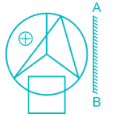Detailed Solution for RRB NTPC Mock Test - 5 (English) - Question 51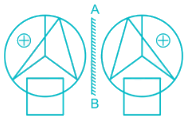Hence, option 3 is the correct alternative.

RRB NTPC Mock Test - 5 (English) - Question 52

In the following question, select the number which can be placed at the sign of question mark (?) from the given alternatives.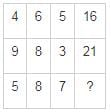Detailed Solution for RRB NTPC Mock Test - 5 (English) - Question 52

The logic here is as follows,

First row: (4 + 6 + 5) + 1 = 16

Second row: (9 + 8 + 3) + 1 = 21

Similarly,

Third row: (5 + 8 + 7) + 1 = 21

Hence, the missing number is 21.

RRB NTPC Mock Test - 5 (English) - Question 53

Of three positive numbers, the ratio of first and second is 4 ∶ 7, that of second and third is 2 ∶ 3. The product of first and third is 4200. What is the sum of the three numbers?

Detailed Solution for RRB NTPC Mock Test - 5 (English) - Question 53

Let the three numbers be x, y and z.

Ratio of x and y = 4 ∶ 7

Ratio of y and z = 2 ∶ 3

Then, x ∶ y ∶ z = 8 ∶ 14 ∶21

Let the three number be 8a, 14a and 21a.

The product of x and z = 4200

⇒ x × z = 4200

⇒ 8a × 21a = 4200

⇒ a2 = 25

⇒ a = 5

First number = x = 8a = 40

Second number = y = 14a = 70

Third number = z = 21a = 105

The sum of three numbers = 40 + 70 + 105 = 215

RRB NTPC Mock Test - 5 (English) - Question 54

What is the simplified form of 412 × 1635 × 6415?

Detailed Solution for RRB NTPC Mock Test - 5 (English) - Question 54

⇒ 412 × 1635 × 6415

⇒ 412 × (42)35 × (43)15

⇒ 412 × 470 × 445

⇒ 412 + 70 + 45

⇒ 4127

RRB NTPC Mock Test - 5 (English) - Question 55

Raja and Kundan together can build a wall in 20 days, Kundan and Mahesh can build the same wall in 30 days and Mahesh and Raja can build the same wall in 24 days. In how many days can all three of them complete the same wall while working together?

Detailed Solution for RRB NTPC Mock Test - 5 (English) - Question 55

Work done by Raja and kundan in one day = (1/R) + (1/K) = 1/20

Work done by Mahesh and kundan in one day = (1/M) + (1/K) = 1/30

Work done by Raja and Mahesh in one day = (1/R) + (1/M) = 1/24

Adding the above three equations we get,

⇒ 2{(1/R) + (1/K) + (1/M)} = (1/20) + (1/30) + (1/24) = 15/120 = 1/8

⇒ (1/R) + (1/K) + (1/M) = 1/16

Work done by Raja, kundan and Mahesh in one day = (1/R) + (1/K) + (1/M) = 1/16

∴ They will complete the wall in 16 days while working together

RRB NTPC Mock Test - 5 (English) - Question 56

Akash purchased an article at a discount of 40% and sold it to Ram at 40% profit. What is the ratio between the marked price and the price for which he sold?

Detailed Solution for RRB NTPC Mock Test - 5 (English) - Question 56

Let, marked price = Rs. x

Discount = 40%

∴ Cost price = x - x × 40/100 = Rs. 0.6x

Profit = 40%

∴ Selling price,

⇒ 0.6x + 0.6x × 40/100

⇒ 0.84x

∴ Required Ratio,

⇒x ∶ 0.84x

⇒ 100 ∶ 84

⇒ 25 ∶ 21

RRB NTPC Mock Test - 5 (English) - Question 57

The average of 11 numbers is 8. If a number 12 is removed, then what will be the new average?

Detailed Solution for RRB NTPC Mock Test - 5 (English) - Question 57

Average of 11 numbers = 8

∴ Sum of 11 numbers = 11 × 8 = 88

After a number 12 is removed

Sum of remaining 10 numbers = 88 - 12 = 76

∴ Average of remaining 10 numbers

= 76/10

= 7.6

RRB NTPC Mock Test - 5 (English) - Question 58

Which answer figure will complete the pattern in the question figure?Detailed Solution for RRB NTPC Mock Test - 5 (English) - Question 58

According to the given figure:Hence, (4) consist of appropriate figure which matches the pattern of question figure.

RRB NTPC Mock Test - 5 (English) - Question 59

The circumferences of two circles are touching externally. The distance between their centres is 12 cm. The radius of one circle is 7 cm. Find the diameter (in cm) of the other circle.

Detailed Solution for RRB NTPC Mock Test - 5 (English) - Question 59

When two circles touch each other externally, the distance between their centres is equal to the sum of their radiuses,

⇒ Distance = 12 = 7 + Radius of other circle

⇒ Radius of other circle = 12 – 7 = 5

∴ Diameter of other circle = 2(5) = 10 cm

RRB NTPC Mock Test - 5 (English) - Question 60

The speed of a train relative to a moving car is 70km/hr more. The car accelerates and starts moving at a speed 10% more than before. Both now travel for 3 hours and the train covers a total distance of 750 km. How much distance did the car travel?

Detailed Solution for RRB NTPC Mock Test - 5 (English) - Question 60

The train covers 750 km in three hours.

The speed of train = 750/3 = 250 km/hr

Initially the train had a relative speed of 70km/hr to the car.

Initial speed of car = 250 – 70 = 180 km/hr

The speed of car now is increased by 10%.

New speed of car = 180 + 18 = 198km/hr

At this speed the car travels for 3 hrs.

∴ The distance travelled by car = 198 × 3 = 594km

RRB NTPC Mock Test - 5 (English) - Question 61

J, K and L started a business. Twice the investment of J is equal to five times the investment of K, and the investment of K is twice the investment of L. Find the share of J in a profit of Rs. 16800?

Detailed Solution for RRB NTPC Mock Test - 5 (English) - Question 61

Let J’s investment be = X

Let K’s investment be = Y

Let L’s investment be = Z

According to the problem,

⇒ 2X = 5Y

⇒ Y = 2X/5      ---- (1)

According to the problem,

⇒ Y = 2Z     ---- (2)

From (1) and (2) we get,

⇒ 2Z = 2X/5

⇒ Z = X/5     ---- (3)

∴ Ratio of investment of J, K and L

⇒ X : 2X/5 : X/5 = 5 : 2 : 1

∴ J’s share in this profit, = 16800 × (5/8) = 10500

RRB NTPC Mock Test - 5 (English) - Question 62

The monthly salaries of A and B are in the ratio 8 : 9. If they get salary increment of Rs. 4000 each, the ratio of new monthly salaries of A and B becomes 10 : 11. What is the new monthly salary of A?

Detailed Solution for RRB NTPC Mock Test - 5 (English) - Question 62

⇒ Let the monthly salaries of A and B be 8x and 9x respectively

⇒ after increment new salary of A and B be (8x + 4000) and (9x + 4000) respectively

⇒ According to question

⇒ (8x + 4000) / (9x + 4000) = 10 / 11

⇒ 11 ⨯ (8x + 4000) = 10 ⨯ (9x + 4000)

⇒ 88x + 44000 = 90x + 40000

⇒ 2x = 4000

⇒ x = Rs. 2000

∴ New salary of A = 8× 2000 + 4000 = Rs. 20000

RRB NTPC Mock Test - 5 (English) - Question 63

Rahul’s salary is 40% more than Mohit’s salary. If Rahul’s salary is increased by 60% and Mohit’s salary is decreased by 20%, then Rahul’s salary will be how much percentage of Mohit’s salary?

Detailed Solution for RRB NTPC Mock Test - 5 (English) - Question 63

Let Mohit’s original salary be 100

Rahul’s original salary (being 40% more than Mohit’s salary) = 100 + (40% of 100) = 100 + 40 = 140

If Rahul’s salary is now increased by 60%, then Rahul’s new salary = 140 + (60% of 140) = 140 + 84 = 224

If Mohit’s salary is decreased by 20%, then Mohit’s new salary = 100 - (20% of 140) = 100 - 20 = 80

To find Rahul’s salary is how much percentage of Mohit’s salary

⇒ 224 = ? % of 80

⇒ ? = (224/80) × 100% = 2.8 × 100% = 280%

∴ Required Percentage = 280%

RRB NTPC Mock Test - 5 (English) - Question 64

The speed of the boat in still water is 18 km/hr and the speed of the current is 2 km/hr. If A man rows his boat upstream for 3 hours and downstream for 2 hours. Find the total distance travelled by him?

Detailed Solution for RRB NTPC Mock Test - 5 (English) - Question 64

Let speed of boat in still water is SB and speed of current is Sand Speed of boat in upstream and downstream is SU and SD.

Speed of boat in still water S= 18 km/hr

Speed of current, S= 2 km/hr

Speed of boat in upstream, S= 18 - 2 = 16 km/hr

Speed of boat in downstream, S= 18 + 2 = 20 km/hr

∴ Total distance travelled = 3 × 16 + 2 × 20 = 48 + 40 = 88 km

RRB NTPC Mock Test - 5 (English) - Question 65

Calculate the value of 0.77777 + 0.7777 + 0.777 + 0.77 + 0.7 + 0.07

Detailed Solution for RRB NTPC Mock Test - 5 (English) - Question 65

0.77777 + 0.7777 + 0.777 + 0.77 + 0.7 + 0.07 = 3.87247

RRB NTPC Mock Test - 5 (English) - Question 66

Evaluate (3 × 25 ) ÷ 5 - 20 × 4 + 75

Detailed Solution for RRB NTPC Mock Test - 5 (English) - Question 66

Simple multiplication 3 × 25 = 75. Bracket takes priority over division, multiplication, addition or subtraction.

Hence, (3 × 25 ) ÷ 5 - 20 × 4 + 75 = 75 ÷ 5 - 20 × 4 + 75 = 15 - 20 × 4 + 75.

Multiplication takes priority over addition or subtraction.

Hence, 15 - 20 × 4 + 75 = 15 - 80 + 75.

Addition takes priority over subtraction.

Hence, 15 - 80 + 75 = 90 - 80 = 10

RRB NTPC Mock Test - 5 (English) - Question 67

If 347P is divisible by 9, then what is the value of P?

Detailed Solution for RRB NTPC Mock Test - 5 (English) - Question 67

We know that if the sum of digits in a number is divisible by 9 then the number is divisible by 9.

Now 3 + 4 + 7 + P should be divisible by 9.

So, 14 + P should be divisible by 9.

Also P is a single digit number.

The number after 14 which is divisible by 9 is 18.

So P is = 18 - 14 = 4.

RRB NTPC Mock Test - 5 (English) - Question 68

Who among the following sportspersons has/have been conferred with Dronacharya Awards for the year 2018?

1. Clarence Lobo
2. Sukhdev Singh
3. Vijay Sharma

Detailed Solution for RRB NTPC Mock Test - 5 (English) - Question 68

The Dronacharya Award is a sports coaching honour of India. Following sports persons were conferred with Dronacharya Awards for the year 2018:

• Subedar Chenanda Achaiah- Boxing
• Vijay Sharma - Weightlifting
• Srinivasa Rao - Table Tennis
• Sukhdev Singh - Athletics
• Clarence Lobo - Hockey (Life Time)
• Tarak Sinha - Cricket (Life Time)
• Jiwan Sharma - Judo (Life Time)
• V.R. Beedu - Athletics (Life Time)
RRB NTPC Mock Test - 5 (English) - Question 69

Which of the following countries will host the fourth summer youth Olympic Games in 2022?

Detailed Solution for RRB NTPC Mock Test - 5 (English) - Question 69
• In 2022, Senegal will host the fourth Summer Youth Olympic Games.
• Senegal has thus become the first African country to host an Olympic event.
• The International Olympic Committee (IOC) unanimously made the decision in Buenos Aires.
• The 2022 Youth Olympics Games will take place in Senegal between May and June.
RRB NTPC Mock Test - 5 (English) - Question 70

The simple interest on a sum is 5/9 of the principal for 25 years. What is the rate (in percentage) of interest per annum?

Detailed Solution for RRB NTPC Mock Test - 5 (English) - Question 70

Given that,

S.I = 5/9 P

We know that,

S.I = (P × R × T)/100

⇒ 5/9P = (P × R × 25)/100

⇒ 5/9 × 4 = R

∴ R = 20/9%

RRB NTPC Mock Test - 5 (English) - Question 71

A train starts from a place A at 8 : 00 a.m. and arrives at another place B at 1 : 30 p.m. on the same day. If the speed of the train is 30 km/hr, then what will be the distance (in km) covered by the train?

Detailed Solution for RRB NTPC Mock Test - 5 (English) - Question 71

Time taken by train to reach B from A =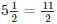hours

Distance travelled by the train = Speed × Time

∴ Distance travelled by the train = 30 × 11/2 = 165 km

RRB NTPC Mock Test - 5 (English) - Question 72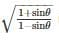is equal to?

Detailed Solution for RRB NTPC Mock Test - 5 (English) - Question 72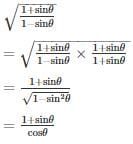RRB NTPC Mock Test - 5 (English) - Question 73

The cost price of 25 books is equal to the selling price of 20 books. The profit percent is

Detailed Solution for RRB NTPC Mock Test - 5 (English) - Question 73

Let the cost price of each book be Rs. 1.

So C. P. of 20 books = Rs. 25
Profit percent =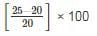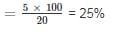RRB NTPC Mock Test - 5 (English) - Question 74

If the profit on selling an article for Rs. 425 is the same as the loss on selling it for Rs. 355, then the cost price of the article is

Detailed Solution for RRB NTPC Mock Test - 5 (English) - Question 74

Let the C. P. of the article be Rs. xx

According to question,

425 - x = x – 355

2x = 425 + 355 = 780

x =780/2

x = 390

RRB NTPC Mock Test - 5 (English) - Question 75

The value of a product depreciates every year at the rate of 10% on its value at the beginning of that year. If the present value of the product is Rs. 7290, its value three years ago was:

Detailed Solution for RRB NTPC Mock Test - 5 (English) - Question 75

Let the required value of product be Rs. y. Then,

Amount before t years, when interest is compounded annually = P(1−R/100)t
y(1−10/100)= Rs.7290
Required value, y = Rs. 10000

RRB NTPC Mock Test - 5 (English) - Question 76

A cartel is ________

Detailed Solution for RRB NTPC Mock Test - 5 (English) - Question 76
• cartel is a group of producers who mutually agree to limit their production, in order to sustain a price floor.
• Cartels are created when a few large producers decide to co-operate with respect to aspects of their market.
RRB NTPC Mock Test - 5 (English) - Question 77

A 13 m ladder is placed against a wall at a distance of 12 m from the foot of the wall. The same ladder is placed against a wall opposite to the previous wall, such that the upper end of the ladder touches the wall 7 m above the previous point. Find the distance between two walls. Given that the foot of the ladder is at the same position in both cases.

Detailed Solution for RRB NTPC Mock Test - 5 (English) - Question 77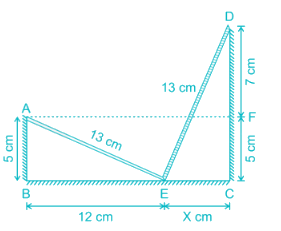In the given figure, apply Pythagoras theorem

AB2 + BE2 = AE2

AB​+ 144 = 169

AB = 5 = CF

Now FD =  7

DC = DF + FC = 5 + 7 = 12

Apply Pythagoras theorem

DC2 + EC2 = DE2

144 + EC= 169

EC = 5 = x

Distance between two walls = 12 + 5 = 17 cm

RRB NTPC Mock Test - 5 (English) - Question 78

Read the following passage and find out the inferences stated through the passage.
With growing industrialisation, it is difficult to reduce air pollution. The world is facing serious problems due to air pollution. While the first world countries have fully developed and have contributed the most to air pollution, the third world countries have yet to develop. It would be unfair to put equal restraints on the third world and the first world countries in order to stop pollution.

Q. Which of the following statements can be inferred from the above passage?

Detailed Solution for RRB NTPC Mock Test - 5 (English) - Question 78

The correct answer is option 1. There should be different restraints on the first world and the third world countries to reduce air pollution.

From the passage, we can conclude that the world is facing serious problems due to air pollution, so we need to control it but same restraints cannot be placed on the first world and the third world countries as it would be unfair to the third world countries. It can be said that there is a need to put different restraints on the third world and the first world countries so as to be fair and to reduce the pollution.Hence, option 1 is the correct solution.

RRB NTPC Mock Test - 5 (English) - Question 79

Rs. 1000 is lent at a 4% p.a. for 1 year at simple interest. If at the end of the first year, the whole amount is lent at compound interest for 2 years, find the total interest (approx.) incurred in these years.

Detailed Solution for RRB NTPC Mock Test - 5 (English) - Question 79

Amount at the end of first year = P + PRT/100 = 1000 + (1000 × 1 × 4)/100 = Rs. 1040
The formula for CI at annual compound interest, including principal sum, is: CI =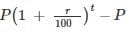Where:

CI = Compound Interest

P = the principal investment amount (the initial deposit or loan amount)

r = the annual interest rate (decimal)

t = the number of years the money is invested or borrowed

CI = 1040(1 + 4/100)2 - 1040

CI = Rs. 84.864

Total interest = 84.864 + 40 = Rs. 124.864 ≈ Rs. 125

RRB NTPC Mock Test - 5 (English) - Question 80

What is the remainder when (256)47 is divided by 15?

Detailed Solution for RRB NTPC Mock Test - 5 (English) - Question 80

We can write, (256)47 = (162)47 = 1694

Now, when 16 is divided by 15, the remainder is 1

∴ When 1694 is divided by 15, the remainder is 1

RRB NTPC Mock Test - 5 (English) - Question 81

Two statements are given followed by two conclusions as responses. You have to consider the statements to be true even if they seem to be at variance from the commonly known facts. You have to decide which of the given conclusion(s) can definitely be drawn from the given statements.

Statements:

1. Swine flu is spread by the outbreak of Influenza virus.
2. The Government is taking a rigorous action to control the spread of Swine flu.

Conclusions:

1. Swine flu is a fatal disease.
2. The Government needs to control the spread of Influenza virus.
Detailed Solution for RRB NTPC Mock Test - 5 (English) - Question 81

Since the disease is caused by the Influenza virus, the government needs to control the spread of the virus to stop the spread of the disease which is stated in conclusion II. Hence conclusion II follows.

There is no information provided in the statement to suggest that the disease is fatal. Therefore, conclusion I cannot be drawn from the given statements and 2 is the right option.

RRB NTPC Mock Test - 5 (English) - Question 82

Statements:

I. Recycling is the best way of effectively managing the generation of waste.

II. The Municipal Corporations in Delhi have decided to collect waste to be recycled.

Conclusions:

I. The amount of waste accumulated in Delhi will reduce.

II. People will help the Municipal Corporations in this initiative.

Detailed Solution for RRB NTPC Mock Test - 5 (English) - Question 82

From the given statements, it can be concluded that recycling will help in managing the accumulation of waste and the Municipal Corporations of the city have decided to get the waste recycled. This will cause a reduction in the amount of waste gathered which is what conclusion I states. Conclusion II states the actions of the people of the city which are not mentioned in the statements.

Therefore, option 1 is the right answer.

RRB NTPC Mock Test - 5 (English) - Question 83

The table given below represents the amount of education loan (in crores) disbursed by 5 banks of a country over 5 years.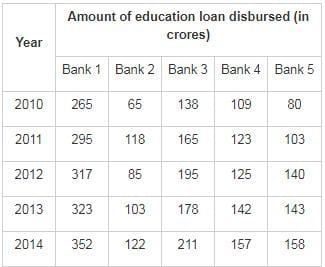Q. What can be said about the two following ratios?
I. Loan amount disbursed by Bank 1 in 2011/Loan amount disbursed by Bank 2 in 2014
II. Loan amount disbursed by Bank 3 in 2014/Loan amount disbursed by Bank 4 in 2011

Detailed Solution for RRB NTPC Mock Test - 5 (English) - Question 83

Loan amount disbursed by Bank 1 in 2011/Loan amount disbursed by Bank 2 in 2014 can be written as

⇒ 295/122

⇒ Its value is around 2.41

Loan amount disbursed by Bank 3 in 2014/Loan amount disbursed by Bank 4 in 2011 can be written as

⇒ 211/123

⇒ Its value is around 1.71

∴ we can say that I > II

RRB NTPC Mock Test - 5 (English) - Question 84

The table given below represents the amount of education loan (in crores) disbursed by 5 banks of a country over 5 years.Q. Which banks show a continuous trend of increase/decrease in loan amount disbursed over 5 years?

Detailed Solution for RRB NTPC Mock Test - 5 (English) - Question 84

From the table we can observe that

For bank 1 the trend is:

It is continuously increasing

For bank 2 the trend is:

Increasing, decreasing, Increasing, Increasing

For bank 3 the trend is:

Increase, increase, decrease, increase

For bank 4 the trend is:

It is continuously increasing

For bank 5 the trend is:

It is continuously increasing

∴ We can say that for bank 1,4 and 5 there is continuous trend of increasing

RRB NTPC Mock Test - 5 (English) - Question 85

The table given below represents the amount of education loan (in crores) disbursed by 5 banks of a country over 5 years.Q. What is the percentage increase in education loan disbursed by Bank 2 from 2010 to 2014?

Detailed Solution for RRB NTPC Mock Test - 5 (English) - Question 85

Given that loan for bank 2 in 2014 is 122 and for year 2010 it is 65

Percentage increase can be given as

⇒ (122 - 65) × 100/65

⇒ 87.69%

∴ Percentage increase in loan is 87.69%

RRB NTPC Mock Test - 5 (English) - Question 86

Average weight of P and Q is 32 kg. Average weight of Q and R is 43 kg. Average weight of P and R is 51 kg. What is the average weight (in kg) of P, Q and R?

Detailed Solution for RRB NTPC Mock Test - 5 (English) - Question 86

∵ Average weight of P and Q is 32 kg

⇒ Sum of their weight = 2 × 32 = 64

⇒ P + Q = 64 kg (1)

Similarly,

Q + R = 86 kg (2)

P + R = 102 kg (3)

Adding equation (1), (2) and (3)

2P + 2Q + 2R = 64 + 86 + 102 = 252

⇒ P + Q + R = 126 kg

∴ Average weight of P, Q and R = (P + Q + R)/3 = 126/3 = 42 kg

RRB NTPC Mock Test - 5 (English) - Question 87

Who was the founder of Saka era?

Detailed Solution for RRB NTPC Mock Test - 5 (English) - Question 87

Saka era is a historical calendar era of ancient India. The beginning of the Shaka era corresponds to the rising of Kanishka I in 78 AD.

RRB NTPC Mock Test - 5 (English) - Question 88

If a + b + c = 45 and ab + bc + ca = 254. Find the value of a2 + b2 + c2 = ?

Detailed Solution for RRB NTPC Mock Test - 5 (English) - Question 88

∵ (a + b + c)2 = a2 + b2 + c2 + 2 (ab + bc + ca)

⇒ (45)2 = a2 + b2 + c2 + 2 × (254)

⇒ 2025 = a2 + b2 + c2 + 508

⇒ a2 + b2 + c2 = 2025 – 508

∴ a2 + b2 + c2 = 1517

RRB NTPC Mock Test - 5 (English) - Question 89

Harisena was the court poet of which king?

Detailed Solution for RRB NTPC Mock Test - 5 (English) - Question 89

Harisena was the court poet of Samudragupta. He was a 4th - century Sanskrit poet, panegyrist, and government minister.

RRB NTPC Mock Test - 5 (English) - Question 90

Rahul and Ravi undertook to do a piece of work for Rs. 9000. Rahul alone could do the work in the 16 days and Ravi in 24 Days. With the assistance Kamlesh, they finished the work in 8 days. How much money should be given to Kamlesh?

Detailed Solution for RRB NTPC Mock Test - 5 (English) - Question 90

⇒ Work done by Rahul in 8 days = 8 × (1/16) = 1/2

⇒ Work done by Ravi in 8 days = 8 × (1/24) = 1/3

It means, Rahul and Ravi done 1/2 and 1/3rd of work, respectively, in 8 days

⇒ Work done by kamlesh = {1 – (1/2 + 1/3)} = 1 – 5/6 = 1/6

⇒ Rahul’s share: Ravi’s share: Kamlesh’sshare = 1/2 : 1/3 : 1/6 = 3 : 2 : 1

⇒ Kamlesh’s share = (1/6) × 9,000 = 1500

∴ Kamlesh’s share is Rs. 1500

RRB NTPC Mock Test - 5 (English) - Question 91

What is the 4th proportional of 3, 8, 12,?

Detailed Solution for RRB NTPC Mock Test - 5 (English) - Question 91

We know that,

a : b :: c : x

Then fourth proportional x = (bc)/a

From the given data,

∴ Fourth proportional x = (8 × 12)/3 = 32

RRB NTPC Mock Test - 5 (English) - Question 92

‘Statue of Liberty’ is the National Emblem of

Detailed Solution for RRB NTPC Mock Test - 5 (English) - Question 92

The Statue of Liberty stands in Upper New York Bay, a universal symbol of freedom. Originally conceived as an emblem of the friendship between the people of France and the U.S. and a sign of their mutual desire for liberty

RRB NTPC Mock Test - 5 (English) - Question 93

If (x + a) is the H.C.F of 2x2 + 5x – 12 and x2 + x – 12 then find the value of a.

Detailed Solution for RRB NTPC Mock Test - 5 (English) - Question 93

Factorising (2x2 + 5x - 12)

2x2 + 5x - 12 = 2x2 + 8x - 3x - 12 = (x + 4) (2x - 3)

Facrtorising (x2 + 5x - 12)

x2 + x - 12 = x2 + 4x - 3x - 12 = (x + 4) (x - 3)

The HCF of (2x2 + 5x - 12) and (x2 + x - 12) = (x + 4)

On comparing (x + 4) and (x + a),

∴ The value of a is 4

RRB NTPC Mock Test - 5 (English) - Question 94

If the standard deviation of a population is 6.5, what would be the population variance?

Detailed Solution for RRB NTPC Mock Test - 5 (English) - Question 94

Given standard deviation σ = 6.5

We know that variance is the square of the standard deviation

∴ Variance = (standard deviation)2 = (σ)2 = 6.52 = 42.25

RRB NTPC Mock Test - 5 (English) - Question 95

Which of the following stations has been renamed to 'Prabhadevi'?

Detailed Solution for RRB NTPC Mock Test - 5 (English) - Question 95

The famed Chhatrapati Shivaji Terminus and headquarters of the Central Railway has been renamed by adding 'Maharaj. Western Railway's Elphinstone Road station has been rechristened as 'Prabhadevi' station. The new name 'Prabhadevi' is dedicated to Goddess Prabhavati Devi, whose 12th century idol is installed in the modern temple, now over three centuries old.

RRB NTPC Mock Test - 5 (English) - Question 96

Find the greatest number that will divide 390, 495 and 300 without leaving a remainder.

Detailed Solution for RRB NTPC Mock Test - 5 (English) - Question 96

Lets factorize each one them

390 = 2 × 3 × 5 × 13

300 = 2 × 2 × 3 × 5 × 5

495 = 3 × 3 × 5 × 11

Hence we can see only two numbers common two all three are 3 × 5 = 15

∴ 15 is the greatest number that divides 390, 495 and 300 without leaving a remainder.

RRB NTPC Mock Test - 5 (English) - Question 97

The numerator and denominator of the fraction are in the ratio 15 : 8, if the numerator of the fraction is decreased by 9 and the denominator is increased by 6 their division gives a quotient of 1.5. Find the fraction.

Detailed Solution for RRB NTPC Mock Test - 5 (English) - Question 97

Let the fraction be (x/y). According to the question, x/y = 15/8      ----(I)

Now if x is decreased by 9 and y is increased by 6 then the fraction is (x - 9)/(y + 6)      ----(II)

According to the problem (x - 9)/(y + 6) = 1.5 = 3/2      ----(III)

From Equation (I), x = (15/8)y      ----(IV)

Putting (IV) in Equation (III) we get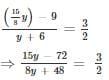⇒ y = 48

Since y = 48 from Equation (IV)  x = (15/8) × 48 = 90

∴ Fraction is 90/48.

RRB NTPC Mock Test - 5 (English) - Question 98

In a two-digit number, the digit at the unit’s place is 1 less than twice the digit at the ten’s place. If the places of both digits are interchanged, the new number formed is 27 more than the original number. The original number is

Detailed Solution for RRB NTPC Mock Test - 5 (English) - Question 98

Let the ten’s digit and unit digit of the two-digit number be x and y respectively.

⇒  Two digit number = 10x + y

According to the given information,

y = 2x– 1

⇒ 2x – y = 1 …………………..(i)

If the places of the digits are interchanged,

10y + x = 10x + y + 27

⇒ 9y -9x = 27

y – x = 3 …………………..(ii)

We get

y = 7

x = 4

∴ The number is 47.

RRB NTPC Mock Test - 5 (English) - Question 99

Which of the following words do not exist in the Preamble of the Indian Constitution?

Detailed Solution for RRB NTPC Mock Test - 5 (English) - Question 99

The Preamble was in the news recently because of the word “Secular” which was added to it in 1976 under the 42nd Amendment Act. The other words that were added to the Preamble were “Socialist” and “Integrity” under the same amendment act.

RRB NTPC Mock Test - 5 (English) - Question 100

Which of the following articles prohibits discrimination on the grounds of religion, race, sex, caste?

Detailed Solution for RRB NTPC Mock Test - 5 (English) - Question 100

Article 15 prohibit discrimination on grounds of religion ,race, sex, or place of birth. Article 16 provides for Equality of opportunity in matters of public employment. Article 17 provides for Abolition of Untouchability and Article 18 provides for Abolition of titles.

## RRB NTPC Mock Tests Series

20 tests
 Use Code STAYHOME200 and get INR 200 additional OFF Use Coupon Code
Information about RRB NTPC Mock Test - 5 (English) Page
In this test you can find the Exam questions for RRB NTPC Mock Test - 5 (English) solved & explained in the simplest way possible. Besides giving Questions and answers for RRB NTPC Mock Test - 5 (English), EduRev gives you an ample number of Online tests for practice

20 tests